Instructions

For the following questions answer them individually

Question 11

# A monochromatic light is incident from air on a refracting surface of a prism of angle $$75^\circ$$ and refractive index $$n_0 = \sqrt 3$$. The other refracting surface of the prism is coated by a thin film of material of refractive index n as shown in figure. The light suffers total internal reflection at the coated prism surface for an incidence angle of $$\theta \leq 60^\circ$$. The value of $$n^2$$ is ___________ .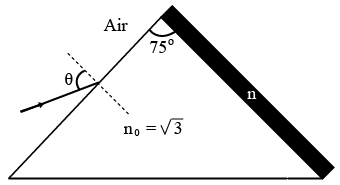Backspace
789
456
123
0.-
Clear AllQuestion 12

# A perfectly reflecting mirror of mass M mounted on a spring constitutes a spring-mass system of angular frequency $$Ω$$ such that $$\frac{4 \pi M Ω}{h} = 10^{24} m^{-2}$$ with h as Planck’s constant. N photons of wavelength $$\lambda = 8 \pi \times 10^{-6} m$$ strike the mirror simultaneously at normal incidence such that the mirror gets displaced by $$1 \mu m.$$ If the value of N is $$x \times 10^{12},$$ then the value of x is ________ .[Consider the spring as mass less]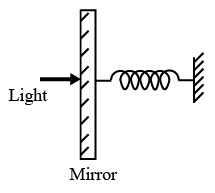Backspace
789
456
123
0.-
Clear AllQuestion 13

# Suppose a $$_{88}^{226} Ra$$ nucleus at rest and in ground state undergoes $$\alpha-$$decay to a $$_{86}^{222} Rn$$ nucleus in its excited state. The kinetic energy of the emitted $$\alpha$$ particle is found to be 4.44 MeV. $$_{86}^{222} Rn$$ nucleus then goes to its ground state by $$\gamma-$$decay. The energy of the emitted $$\gamma$$ photon is ________ ke V.[Given: atomic mass of $$_{88}^{226} Ra = 226.005 u,$$ atomic mass of $$_{86}^{222} Rn = 222.000 u,$$ atomic mass of $$\alpha$$ particle = 4.000 u, $$1 u = 931 Me V/c^2,$$ c is speed of the light]

Backspace
789
456
123
0.-
Clear AllQuestion 14

# An optical bench has 1.5 m long scale having four equal divisions in each cm. While measuring the focal length of a convex lens, the lens is kept at 75 cm mark of the scale and the object pin is kept at 45 cm mark. The image of the object pin on the other side of the lens overlaps with image pin that is kept at 135 cm mark. In this experiment, the percentage error in the measurement ofthe focal length ofthe lens is _____________ .

Backspace
789
456
123
0.-
Clear AllInstructions

A musical instrument is made using four different metal strings, 1, 2, 3 and 4 with mass per unit length $$\mu, 2\mu, 3\mu$$ and $$4\mu$$ respectively. The instrument is played by vibrating the strings by varying the free length in between the range $$L_0$$ and $$2L_0$$. It is found that in string-1 $$(\mu)$$ at free length $$L_0$$ and tension $$T_0$$ the fundamental mode frequency is $$f_0$$.
List-I gives the above four strings while list-II lists the magnitude of some quantity.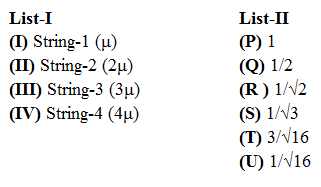Question 15

# If the tension in each string is $$T_0$$, the correct match for the highest fundamental frequency in $$f_0$$ units will be,Question 16

# The length of the strings 1, 2, 3 and 4 are kept fixed at $$L_0, \frac{3L_0}{2}, \frac{5L_0}{4}$$ and $$\frac{7L_0}{4}$$, respectively. Strings 1, 2, 3, and 4 are vibrated at their $$1^{st}, 3^{rd}, 5^{th}$$ and $$14^{th}$$ harmonics, respectively such that all the strings have same frequency. The correct match for the tension in the four strings in the units of $$T_0$$ will be,Instructions

In a thermodynamic process on an ideal monatomic gas, the infinitesimal heat absorbed by the gas is given by T $$\triangle X,$$ where T is temperature of the system and $$\triangle X$$ is the infinitesimal change in a thermodynamic quantity X of the system. For a mole of monatomic ideal gas $$X = \frac{3}{2} R ln \left(\frac {T}{T_A}\right) + R ln \left(\frac {V}{V_A}\right)$$ Here, R is gas constant, V is volume of gas, $$T_A$$ and $$V_A$$ are constants.
The List-I below gives some quantities involved in a process and List-II gives some possible values of these quantities.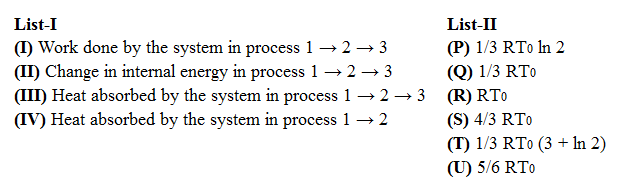Question 17

# If the process carried out on one mole of monatomic ideal gas 1s as shown in figure in the PV -diagram with $$P_0 V_0 = \frac{1}{3} RT_0$$ the correct match is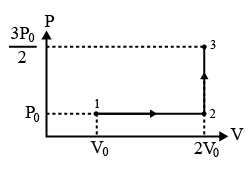Question 18

# If the process on one mole of monatomic ideal gas 1s as shown in the TV-diagram with $$P_0 V_0 = \frac{1}{3} RT_0$$ the correct match is,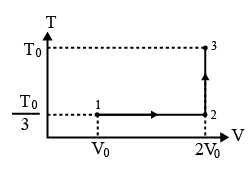Instructions

For the following questions answer them individually

Question 19

# The cyanide process of gold extraction involves leaching out gold from its ore with $$CN^{-}$$ in the presence of Q in water to form R. Subsequently, R is treated with T to obtain Au and Z. Choose the correct option(s)Question 20

# With reference to aqua regia, choose the correct option(s)OR